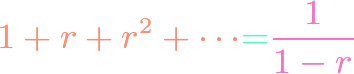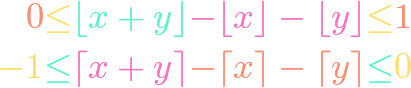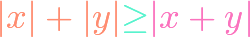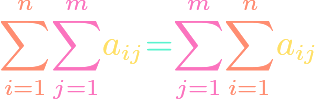# Algebra## Geometric Series

https://www.youtube.com/watch?v=wqHFq1OqmD4 Where an arithmetic series adds a common difference to each successive term, a geometric series multiplies successive terms by a common ratio. We show how to evaluate such a series, both in its finite and infinite variants. The infinite variant is one of the simplest infinite series that actually converge, and its formula is …## Floor and Ceiling Sum Bounds

https://www.youtube.com/watch?v=EEI6ucn5FS8 We show the analogue of the triangle inequality when floor and ceiling functions replace absolute value. This results in some interesting inequalities that are useful in obtaining approximation results. The floor function one is $$0le lfloor x+y rfloor -lfloor x rfloor – lfloor y rfloor le 1.$$## Real Triangle Inequality

https://www.youtube.com/watch?v=mUhBemtfivQ The triangle inequality $$|x|+|y|ge |x+y|$$ for real numbers is an indispensable tool in mathematical analysis, otherwise known as calculus, because it allows us to approximate quantities by bounds. We prove a general version of it using the ordinary absolute value and for any number of variables.## Compound vs. Simple Interest

https://www.youtube.com/watch?v=aLbfdbiAkXg Common knowledge says that banks give us a better deal by using compound interest instead of simple interest. Even though there are forces working in both directions, this is indeed true. This fact is equivalent to Bernoulli’s inequality. We also show that the AM-GM inequality can be used to prove that compounding more times …## Discrete Fubini’s Principle

https://www.youtube.com/watch?v=t_sZk0G_5w4 Sums and products can be written compactly using $sum$ and $prod$ notation, but it is sometimes possible to write the same sum or product in more than one way. The so-called discrete Fubini’s principle is a powerful method of exchanging the order in which the indices occur in a nested sum or product. It …